##### Actions

(diff) ← Older revision | Latest revision (diff) | Newer revision → (diff)

A submoduleof the direct sum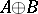of two modulesandover some ring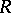. An additive relation can thus be regarded also as a (not necessary single-valued) mapping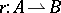or, more exactly, as a "many-valued" homomorphism, i.e. a homomorphism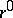of the submoduleinto the quotient modulewhereHere,is the relation inverse to; it consists of all pairs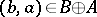such that. Conversely, if a submodule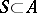, a quotient moduleof the moduleand a homomorphismare given, then there also exists a unique additive relationsuch that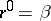.

If two additive relationsand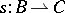are given, then, as in the case of other binary relations, it is possible to define their product,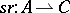, which is the set of all pairs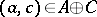such that there exists an elementfor which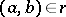and. This multiplication is associative (wherever defined) and, moreover, the additive relations form a category with involution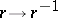.

Additive relations are used in natural definitions of connecting homomorphisms for exact sequences of complexes. The above considerations are valid not only in the category of modules, but also in any other Abelian category.

How to Cite This Entry: